# 为什么大量的if else这么不受待见？怎么“干掉”它？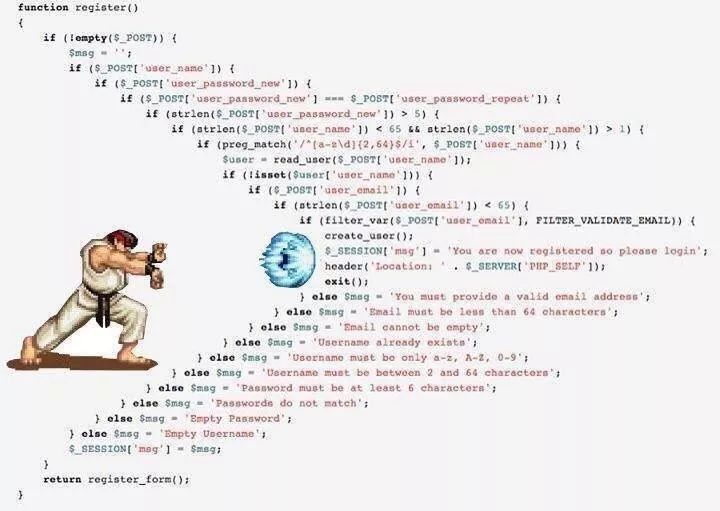if else与switch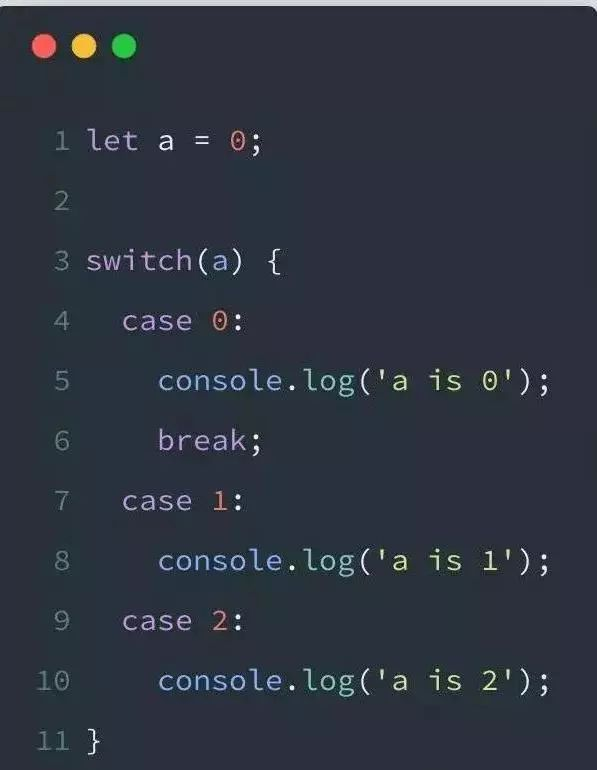OK，熟练的程序猿应该已经发现Bug所在了，在第8行和第10行下面我没有添加关键字break; 这就导致这段代码的行为逻辑与我的设计初衷不符了。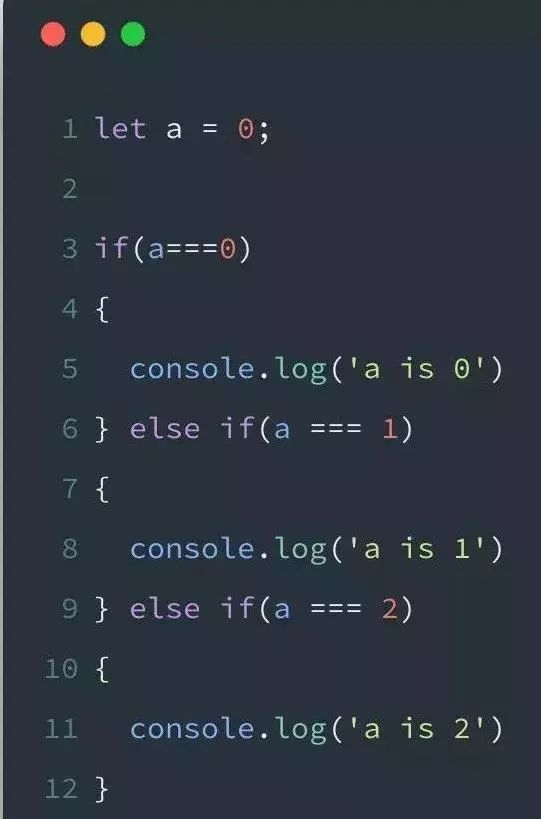switch尽管对于break很宽容，但是对判断条件很严苛，case后面只能跟常量，如果你用C编写的话，甚至只能用int类型作为判断条件。对于我们这么潇洒自如的程序猿来说，这种限制实在是太麻烦了，用if的话，别说是常量了，我用函数都可以，真正做到方便快捷。

switch的优点

# 项目中的if else太多了，该怎么重构？

if else过多的话，一般都是用策略模式来进行重构，策略模式也非常的简单。先定义一个接口，各种处理分支实现这个接口，定义好 条件->处理类的映射关系，然后根据条件找到响应的处理类执行即可，当有新的分支的话，只需要增加一个接口实现类，增加一个条件->映射类的映射关系即可。还是很好容易理解的

# 介绍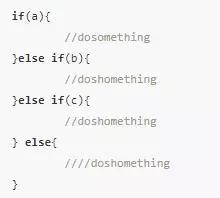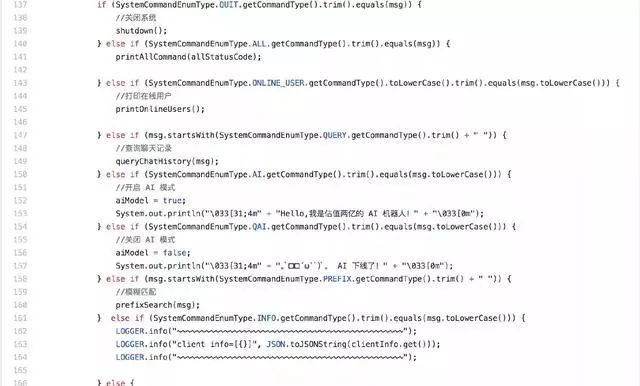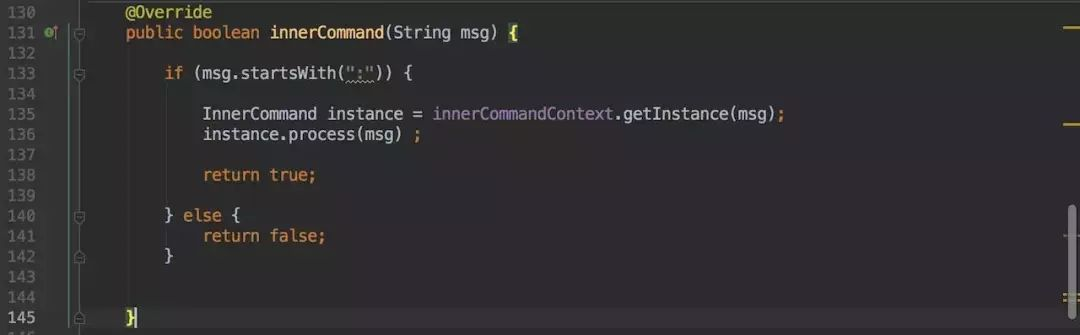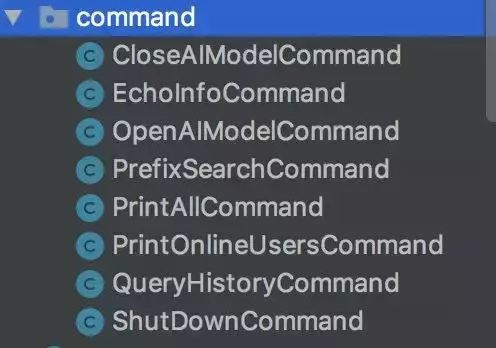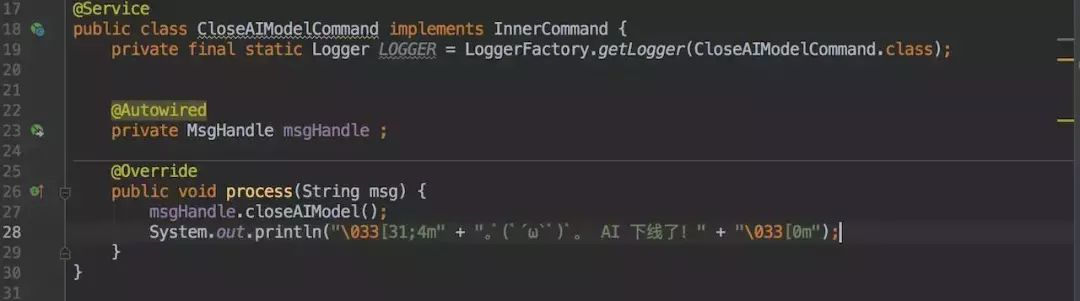# 实现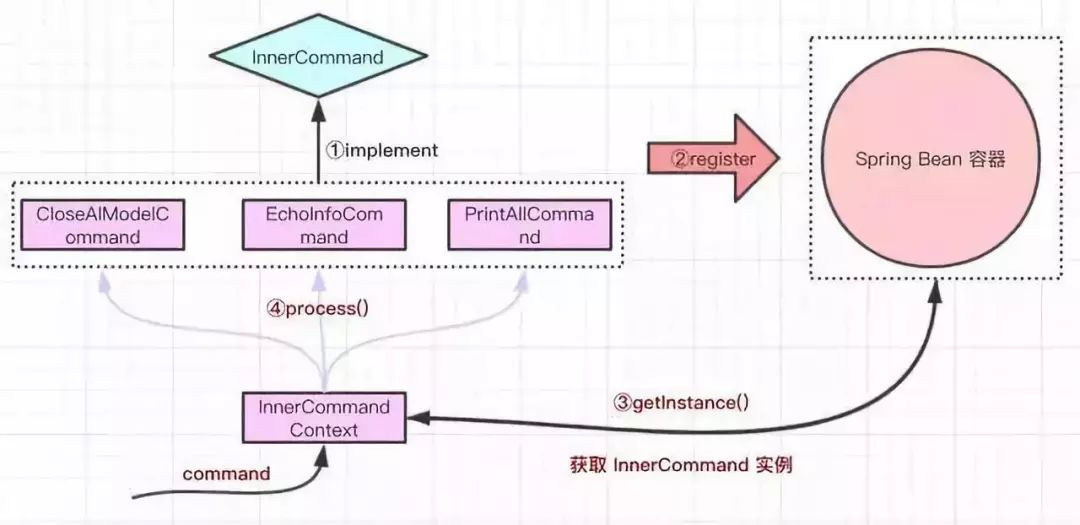• 定义一个 InnerCommand 接口，其中有一个 process 函数交给具体的业务实现。

• 根据自己的业务，会有多个类实现 InnerCommand 接口；这些实现类都会注册到 Spring Bean 容器中供之后使用。

• 通过客户端输入命令，从 Spring Bean 容器中获取一个 InnerCommand 实例。

• 执行最终的 process 函数。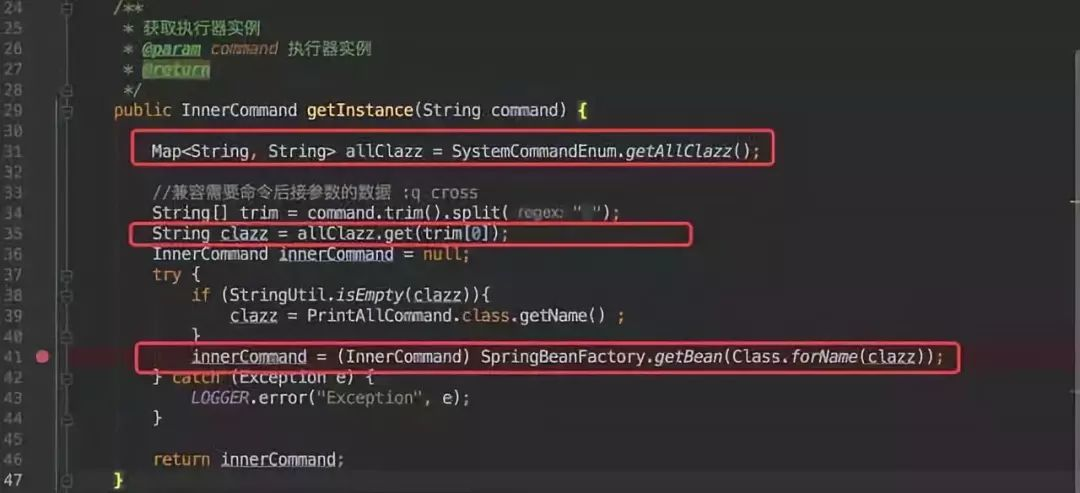• 第一步是获取所有的 InnerCommand 实例列表。

• 根据客户端输入的命令从第一步的实例列表中获取类类型。

• 根据类类型从 Spring 容器中获取具体实例对象。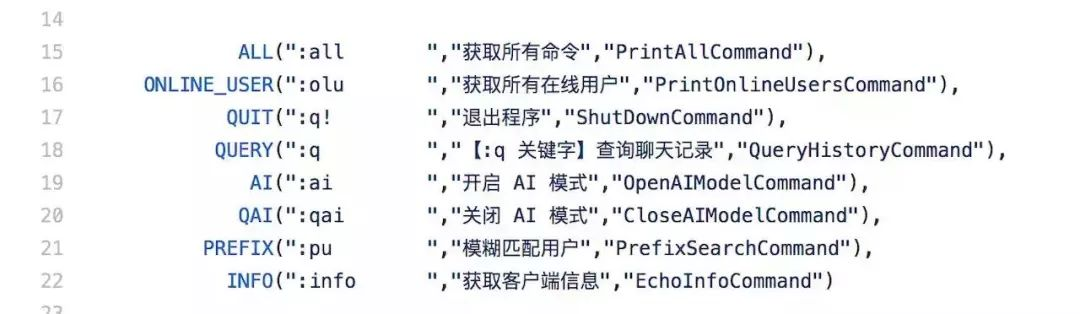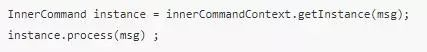# 总结

github地址：https://github.com/crossoverJie/cim

# 1. 最简单的计算器实现(示例1)

public class Main { public static void main(String[] args) { // 计算类型：1：加法 2：减法 3：乘法 4：除法 // 计算类型和计算的值都是正常客户端传过来的 int calcType = 1; int num1 = 1; int num2 = 3;  // 计算器真正的实现 int result = 0; if (calcType == 1) { result = num1 + num2; } else if (calcType == 2) { result = num1 - num2; } else if (calcType == 3) { result = num1 * num2; } else if (calcType == 4) { result = num1 / num2; } System.out.println(result); }}



1. 计算类型使用了魔法值(就是写死的值)，程序中应该避免直接使用魔法值，可以使用枚举类来优化；

2. 计算器功能的每种操作非常简单，只需要对两个数字运算一下就完了，每个操作也就是一行代码的事，但实际开发过程中每种业务处理可能要几十行甚至更多行代码来完成，现在是4种类型，如果每种类型的业务需要100行代码，这四种就会有400行代码，这只是if else的代码量再加上该方法的其它代码可以想象该方法的代码量很大，我们需要控制方法的行数，一般通常控制到100行以内，我们可以通过将每个运算的具体实现都提取成一个单独的方法，这样一来就缩小每个if else的代码的行数，只需要一行代码，只需要调用一个方法就可以了。

# 2. 枚举类+提取方法实现(示例2)

• 增加一个枚举类CalcTypeEnum用于表示所有的运算类型, 使用枚举类来优化魔法值

• 将每种具体的运算实现提取为独立的方法


public enum CalcTypeEnum { ADD(1, "加法操作"), SUB(2, "减法操作"), MUL(3, "乘法操作"), DIV(4, "除法操作"), ; private Integer code; private String description; CalcTypeEnum(Integer code, String description) { this.code = code; this.description = description; } public Integer getCode() { return code; } public String getDescription() { return description; }}public class Main { public static void main(String[] args) { // 计算类型：1：加法 2：减法 3：乘法 4：除法 int calcType = 1; int num1 = 1; int num2 = 3; int result = 0;    // 使用枚举来代替魔法值 if (CalcTypeEnum.ADD.getCode().equals(calcType)) {   // 假如加法运算中还包含其它业务逻辑，那么这些逻辑也被封装到方法中了，此处只有一行的代码量 result = add(num1, num2); } else if (CalcTypeEnum.SUB.getCode().equals(calcType)) { result = sub(num1, num2); } else if (CalcTypeEnum.MUL.getCode().equals(calcType)) { result = mul(num1, num2); } else if (CalcTypeEnum.DIV.getCode().equals(calcType)) { result = div(num1, num2); } System.out.println(result); }  /** 将具体的运算实现代码封装成独立的方法 */ public static int add(int num1, int num2) { System.out.println("加法运算其它业务逻辑start"); System.out.println("此处省略几十行代码..."); System.out.println("加法运算其它业务逻辑end"); return num1 + num2; } public static int sub(int num1, int num2) { return num1 - num2; } public static int mul(int num1, int num2) { return num1 * num2; } public static int div(int num1, int num2) { return num1 / num2; }}



main方法属于客户端，加减乘除功能是具体的功能实现，用来提供服务，我们不能将服务和客户端代码写到一起，每个类的职责应该更明确，即服务提供方用于专门提供服务，客户端调用方用于调用服务。

# 3. 计算器功能单独封装成类(示例3)


public class Calculator { public int calc(CalcTypeEnum calcType, int num1, int num2) { int result = 0; if (CalcTypeEnum.ADD.equals(calcType)) { result = add(num1, num2); } else if (CalcTypeEnum.SUB.equals(calcType)) { result = sub(num1, num2); } else if (CalcTypeEnum.MUL.equals(calcType)) { result = mul(num1, num2); } else if (CalcTypeEnum.DIV.equals(calcType)) { result = div(num1, num2); } return result; } public int add(int num1, int num2) {   System.out.println("加法运算其它业务逻辑start"); System.out.println("此处省略几十行代码..."); System.out.println("加法运算其它业务逻辑end"); return num1 + num2; } public int sub(int num1, int num2) { return num1 - num2; } public int mul(int num1, int num2) { return num1 * num2; } public int div(int num1, int num2) { return num1 / num2; }}



public class Main {
public static void main(String[] args) {
int num1 = 1;
int num2 = 3;
Calculator calculator = new Calculator();
int result = calculator.calc(CalcTypeEnum.ADD, num1, num2);
System.out.println(result);
}
}


1. 枚举类CalcTypeEnum增加乘方的枚举值

2. 修改Calculator类，增加一个乘方的方法，然后在calc方法中再增加一个else if 块

# 4.将具体运算分别封装成单独的类中(示例4)

/**
* 计算策略.
*
*/
public interface CalcStrategy {
int calc(int num1, int num2);
}
/**
* 加法操作
*/
public class AddStrategy implements CalcStrategy {
@Override
public int calc(int num1, int num2) {
System.out.println("加法运算其它业务逻辑start");
System.out.println("此处省略几十行代码...");
System.out.println("加法运算其它业务逻辑end");
return num1 + num2;
}
}
/**
* 减法操作.
*/
public class SubStrategy implements CalcStrategy {
@Override
public int calc(int num1, int num2) {
return num1 - num2;
}
}
/**
* 乘法操作
*/
public class MulStrategy implements CalcStrategy {
@Override
public int calc(int num1, int num2) {
return num1 * num2;
}
}
/**
* 除法操作
*/
public class DivStrategy implements CalcStrategy {
@Override
public int calc(int num1, int num2) {
return num1 / num2;
}
}



public enum CalcTypeEnum { ADD(1, "加法操作"), SUB(2, "减法操作"), MUL(3, "乘法操作"), DIV(4, "除法操作"), // 求余数运算 REM(5, "求余操作"), ;}/** * 求余操作 */public class RemStrategy implements CalcStrategy { @Override public int calc(int num1, int num2) { return num1 % num2; }}public class Main {  // 用户要计算的类型 private static final int CALC_TYPE = 5; public static void main(String[] args) {   // 根据用户要运算的类型调用相应实现类的方法 CalcStrategy calcStrategy = null; if (CalcTypeEnum.ADD.getCode().equals(CALC_TYPE)) { calcStrategy = new AddStrategy(); } else if (CalcTypeEnum.SUB.getCode().equals(CALC_TYPE)) { calcStrategy = new SubStrategy(); } else if (CalcTypeEnum.MUL.getCode().equals(CALC_TYPE)) { calcStrategy = new MulStrategy(); } else if (CalcTypeEnum.DIV.getCode().equals(CALC_TYPE)) { calcStrategy = new DivStrategy(); } else if (CalcTypeEnum.REM.getCode().equals(CALC_TYPE)) { calcStrategy = new RemStrategy(); } int result = calcStrategy.calc(10, 20); System.out.println(result); }}



# 5. 为示例4引入上下文(示例5)


public class CalcStrategyContext {  // 运算接口 private CalcStrategy strategy;  // 通过构造函数或者set方法赋值 public CalcStrategyContext(CalcStrategy strategy) { this.strategy = strategy; } public int executeStrategy(int a, int b) {   // 简单调用具体实现对应的方法 return strategy.calc(a, b); } public CalcStrategy getStrategy() { return strategy; } public void setStrategy(CalcStrategy strategy) { this.strategy = strategy; }}public class Main { private static final Integer CALC_TYPE = 1; public static void main(String[] args) { CalcStrategy calcStrategy = null; if (CalcTypeEnum.ADD.getCode().equals(CALC_TYPE)) { calcStrategy = new AddStrategy(); } else if (CalcTypeEnum.SUB.getCode().equals(CALC_TYPE)) { calcStrategy = new SubStrategy(); } else if (CalcTypeEnum.MUL.getCode().equals(CALC_TYPE)) { calcStrategy = new MulStrategy(); } else if (CalcTypeEnum.DIV.getCode().equals(CALC_TYPE)) { calcStrategy = new DivStrategy(); }    // 这里不再直接调用接口方法了，而是调用上下文类中的方法    // 上下文就是对接口的一种简单的装饰和封装 CalcStrategyContext context = new CalcStrategyContext(calcStrategy); int result = context.executeStrategy(20, 30); System.out.println(result); }}



CalcStrategy#calc

CalcStrategyContext#executeStrategy


/** * 促销上下文 * @author Mengday Zhang * @version 1.0 * @since 2019-08-16 */public class PromotionContext { public BigDecimal executeStrategy() { BigDecimal promotionPrice = null; List<PromotionStrategy> promotionList = ...; for (PromotionStrategy strategy : promotionList) { // 执行下次运算需要将上次运算的结果作为下次运算的参数， // 每次计算的结果需要和传进来的上次运算结果做比较，取最小值作为新的结果返回 promotionPrice = strategy.executeStrategy(promotionPrice); } return promotionPrice; }}



1. 提供一个策略接口

2. 提供多个策略接口的实现类

3. 提供一个策略上下文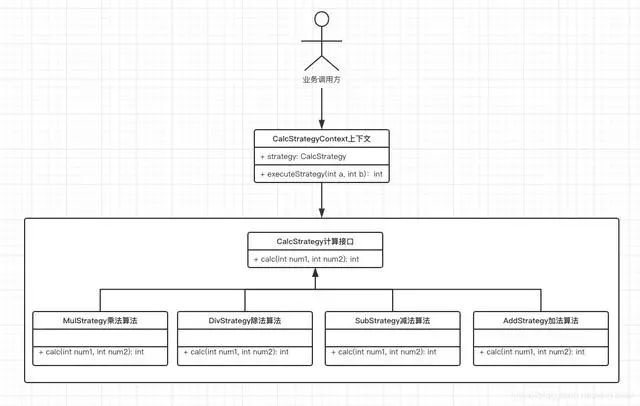• 可以自由切换算法(具体实现)

• 避免了多条件的判断(干掉了if else)

• 扩展性好可以定义新的算法提供给使用者(增加新功能时只需要增加代码而不需要修改代码)

• 算法类数量增多，每个算法都是一个类，这对于初级程序员比较难以接受

# 6. 通过枚举类撤掉干掉if else(示例6)

public enum CalcTypeEnum {   // code 一般设置为具体实现类的前缀 ADD("Add", "加法操作", new AddStrategy()), SUB("Sub", "减法操作", new SubStrategy()), MUL("Mul", "乘法操作", new MulStrategy()), DIV("Div", "除法操作", new DivStrategy()), ; private String code; private String description; private CalcStrategy calcStrategy; CalcTypeEnum(String code, String description, CalcStrategy calcStrategy) { this.code = code; this.description = description; this.calcStrategy = calcStrategy; }  // 根据code获取对应的枚举类型 public static CalcTypeEnum getCalcTypeEnum(String code) { for (CalcTypeEnum calcTypeEnum : CalcTypeEnum.values()) { if (calcTypeEnum.getCode().equals(code)) { return calcTypeEnum; } } return null; } public String getCode() { return code; } public String getDescription() { return description; } public CalcStrategy getCalcStrategy() { return calcStrategy; }}public class Main { private static final String CALC_TYPE = "Sub"; public static void main(String[] args) { // 消除if else，根据code获取到对应的枚举类，进而获取到对应的计算实现类 CalcStrategy addStrategy = CalcTypeEnum.getCalcTypeEnum(CALC_TYPE).getCalcStrategy(); CalcStrategyContext context = new CalcStrategyContext(addStrategy); int result = context.executeStrategy(20, 30); System.out.println(result); }}



# 7. 通过反射彻底干掉if else(方式二)


/** * 加法操作 */public class AddStrategy implements CalcStrategy { private static AddStrategy addStrategy; private AddStrategy() { }  // 懒汉模式实现单例类 public static AddStrategy getInstance() { if (addStrategy == null) { addStrategy = new AddStrategy(); } return addStrategy; } @Override public int calc(int num1, int num2) {   System.out.println("加法运算其它业务逻辑start"); System.out.println("此处省略几十行代码..."); System.out.println("加法运算其它业务逻辑end"); return num1 + num2; }}



public class CalcStrategyUtils {
public static CalcStrategy getCalcStrategy(String calcType) {
try {
// 这里包名是写死的，开发时需要将实现类统一放到该包下面，类的命名也是有规则的，以Strategy作为后缀
String path = "com.example.design.strategy.demo7." + calcType.concat("Strategy");
Class<?> clazz = Class.forName(path);
CalcStrategy instance = (CalcStrategy) clazz.getDeclaredMethod("getInstance").invoke(null, null);
return instance;
} catch (Exception e) {
e.printStackTrace();
throw new RuntimeException("Load [" + calcType.concat("Strategy") + "] Error :", e);
}
}
}
public class Main {
private static final String CALC_TYPE = "Add";
public static void main(String[] args) {
// 通过反射获取到具体实现类型，从而消除if else
CalcStrategy addStrategy = CalcStrategyUtils.getCalcStrategy(CALC_TYPE);
CalcStrategyContext context = new CalcStrategyContext(addStrategy);
int result = context.executeStrategy(20, 30);
System.out.println(result);
}
}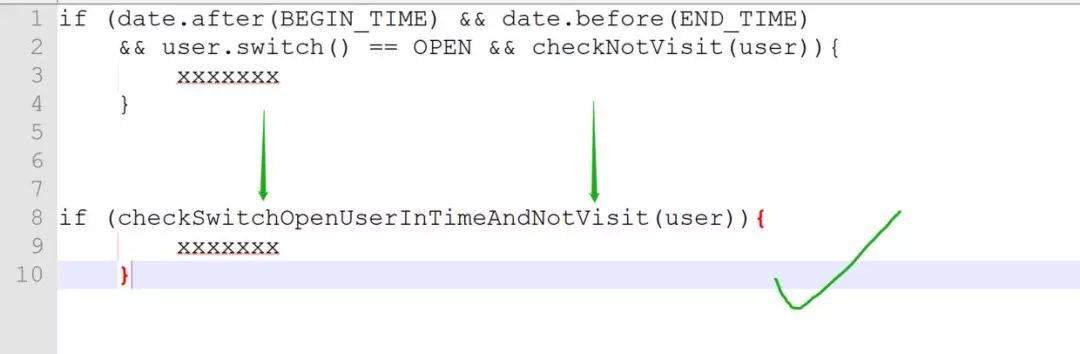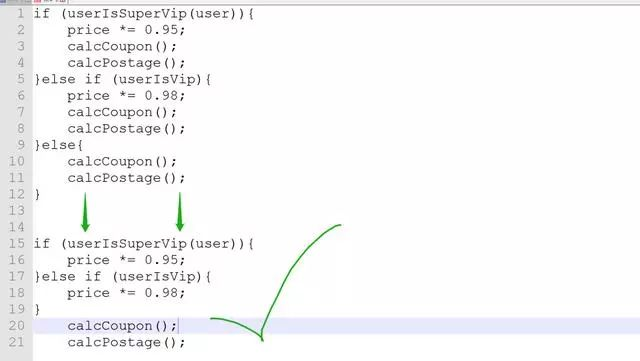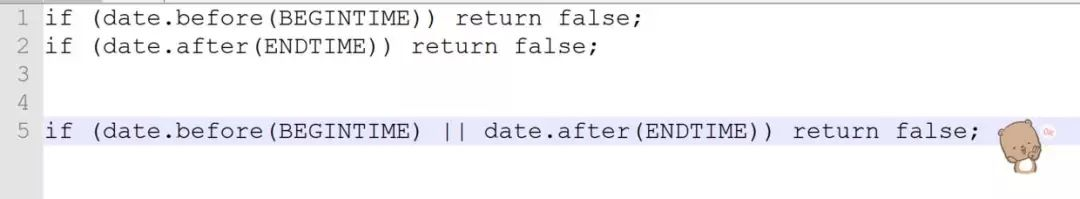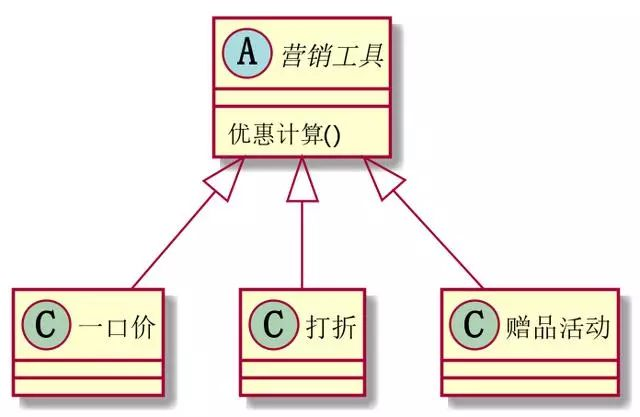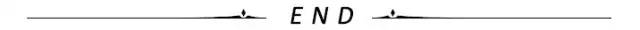（点击标题可跳转阅读）

## 10 个常用的软件架构模式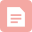博客

12-13879博客
【技术让梦想更伟大】专辑 | 原创汇总
12-08880博客
arm-none-eabi-gcc 源码编译 toolchain 构建过程
09-2412博客
UDP 分片 与 丢包，UDP 真的比 TCP 高效吗？
09-2314博客
udp如何实现可靠性传输？（附开源项目）
09-2117博客

09-2031博客
Cortex-M中特别实用的DWT计数器
09-1921博客

09-1812博客
AVR单片机与C语言的一些入门简要概述
09-17196博客

09-17193博客
INTEL HEX FILES EXPLAINED
09-1715博客
VS code搭建 C 和 C++ 环境的完整图文教程！
09-16150博客

09-1335博客

09-1231博客
OOPC开发从未如此简单!
09-0735博客

09-0798博客
stm32CubeIDE中CMSIS_V1和CMSIS_V2选项的区别
09-06648博客
9个嵌入式硬件转软件小技巧
09-0653博客
09-0426博客
C语言接口与实现:创建可重用软件的技术
09-04185

### “相关推荐”对你有帮助么？

•非常没帮助
•没帮助
•一般
•有帮助
•非常有帮助被折叠的  条评论 为什么被折叠?到【灌水乐园】发言点击重新获取扫码支付1.余额是钱包充值的虚拟货币，按照1:1的比例进行支付金额的抵扣。
2.余额无法直接购买下载，可以购买VIP、C币套餐、付费专栏及课程。余额充值